# Electronics and Communication Engineering - Power Electronics

### Exercise :: Power Electronics - Section 8

6.

A crowbar circuit is generally needed for protection under fault conditions.

 A. True B. False

Explanation:

No answer description available for this question. Let us discuss.

7.

Forward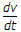rating of an SCR is

 A. the maximum rate of rise of anode voltage which will trigger the SCR B. the maximum rate of rise of anode voltage which will not trigger the SCR if gate signal is applied C. the maximum rate of rise of anode voltage which will not trigger the SCR if gate signal is not applied D. either (b) or (c)

Explanation:

No answer description available for this question. Let us discuss.

8.

The number of leads in a DIAC are

 A. 2 B. 3 C. 4 D. 6

Explanation:

No answer description available for this question. Let us discuss.

9.

The condition for underdamped oscillations in a series RLC circuit is

 A.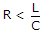B.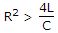C.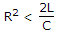D.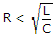Explanation:

No answer description available for this question. Let us discuss.

10.

The method of triggering SCS is

 A. application of positive pulse at cathode gate or negative pulse at anode gate B. application of pulse at anode gate C. application of pulse at cathode gate D. application of low voltage at anode